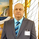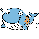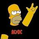# [RS][NM]Improved Linear Regression Bull and Bear Power v01

9551 views
9551
The base code for this indicator was created by RicardoSantos
What I added is a signal line that indicates when to buy and when to sell.

Combine with a zero-lag indicator like ZeroLagEMA_LB by LazyBear (suggested period = 34)
Then use the following Rules of engagement :
Current price > ZLEMA & Signal line of BBP_NM is green : BUY
Current price < ZLEMA & Signal line of BBP_NM is red : SELL Please click the like button if you dig this indicator !
Comment: I have found a little calculation error in this script where if there is no data for either bull or bear volume, the indicator will not plot. This has been corrected in version 2
```//@version=1
// this code uses the Linear Regression Bull and Bear Power indicator created by RicardoSantos
// and adds a signal line
// Use : if signal line is changes color, you have your signal, green = buy, red = sell
// Advice : best used with a zero lag indicator like ZeroLagEMA_LB from LazyBear
// if price is above ZLEMA and signal = green => buy, price below ZLEMA and signal = red => sell
study(title='[RS][NM]Improved Linear Regression Bull and Bear Power v01', shorttitle='BBP_NM', overlay=false)
window = input(title='Lookback Window:', type=integer, defval=10)

f_exp_lr(_height, _length)=>
_ret = _height + (_height/_length)

h_value = highest(close, window)
l_value = lowest(close, window)

h_bar = n-highestbars(close, window)
l_bar = n-lowestbars(close, window)

bear = 0-f_exp_lr(h_value-close, n-h_bar)
bull = 0+f_exp_lr(close-l_value, n-l_bar)
direction = bull*2 + bear*2

plot(title='Bear', series=bear, style=columns, color=maroon, transp=90)
plot(title='Bull', series=bull, style=columns, color=green, transp=90)
plot(title='Direction', series=direction, style=line, linewidth=3, color= direction > 0 ? green : red)```
Cut your hidden banking costs !
https://revolut.com/r/nicoqbx0f_exp_lr(_height, _length)=>
_ret = _height + (_height/_length) Any other way to do this in pinescript?On trying to learn this language, what the n stands for, also the symbol => on the following piece of code: f_exp_lr(_height, _length)=>
_ret = _height + (_height/_length). Thanks.syracusepro
@syracusepro, sorry for the late reply
n returns the bar number (a built-in variable
the => symbol declares f_exp_lr as a function that requires 2 parameters, height and length and using those parameters calculates the return (_ret) using the formula height + (_height/_length)

This allows to create the calculation formula only once and use it with different parameters throughout the scripts as you can see :
bear = 0-f_exp_lr(h_value-close, n-h_bar)
bull = 0+f_exp_lr(close-l_value, n-l_bar)

hope this helps ... and again sorry for the (very) late replyloujill
Hi loujill, thanks for your request. I do not think it is a good idea to code a strategy for this indicator as it depends a lot on your trading style, the risk you are prepared to take, the targets you are looking for and the time frame you are trading. The challenge is on your side to find ways this indicator can harvest some pips for you ;)